Next: Accuracy Up: Implementation Previous: The order   Contents

Storage

The boxes generated by the bisection process are stored in an interval matrix:

Box_Solve_General_Interval(M,m)
The algorithm try to manage the storage in order to solve the problem with the given number M. As seen in section 2.3.1.2 two storage modes are available, the Direct Storage and the Reverse Storage modes, which are obtained by setting the global variable Reverse_Storage to 0 (the default value) or at least to the number of unknowns plus 1. See also section 8.3 to use a mixed strategy between the direct and reverse mode.

For both modes the algorithm will first run until the bisection of the current box leads to a total number of boxes which exceed the allowed total number. It will then delete the boxes in the list which have been already bisected, thereby freeing some storage space (usually larger for the reverse mode than for the direct mode) and will start again.

If this is not sufficient the algorithm will consider each box in the list and determine if the bisection process applied on the box does create any new boxes otherwise the box is deleted from the list. Note that this procedure is computer intensive and constitute a "last ditch" effort to free some storage space. You can disable this feature by setting the integer variable Enable_Delete_Fast_Interval to 0. If the storage space freed by this method is not sufficient the algorithm will exit with a failure return.

If epsilonf=0, epsilon=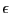and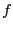is the largest width of the intervals in TestDomain, then the number of boxes that will be considered in the direct mode is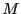with, in the worst case: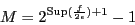(2.1)

where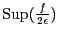is the largest integer greater than. In the direct storage mode the storage space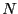will be in the worst case: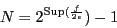(2.2)

In the reverse storage mode the storage space is only: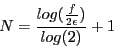(2.3)

Note that with the reverse storage mode, storage is not really a problem. For example if the width of the initial box is 1000 and the accuracy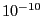, then the necessary storage space is only 44. Thus only the computation time or the conditioning of the functions may lead to a failure of the algorithm. If epsilonf is not equal to 0 the size of the storage cannot be estimated.

If the procedure has to be used more than once it is possible to speed up the computation by allocating the storage space before calling the procedure. Then you may indicate that the storage space has been allocated beforehand by indicating a negative value for M, the number of boxes being given by the absolute value of M.

Note also that the bisection process applied only to one variable may lead to a better estimation of the roots of the system if the algorithm stops when the accuracy required on the variable is reached: indeed, compared to the standard algorithm, one (or more) of the variable may have been individually split before reaching the step where a full bisection will lead to a solution (see the example in section 2.4.3.2).

Note also a specific use of ALIAS_RANDG: if this integer is not set to 0, then every ALIAS_RANDG iteration the algorithm will put the box having the largest width as current box, except if the number of boxes remaining to be processed is greater than half the total number of available boxes.Next: Accuracy Up: Implementation Previous: The order   Contents
Jean-Pierre Merlet 2012-12-20